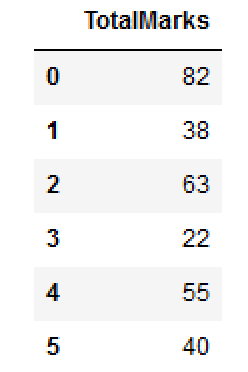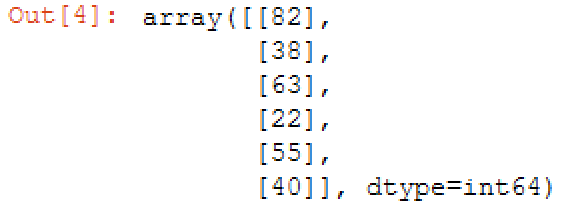Net-informations.com

# Convert Pandas DataFrame to NumPy Array

The NumPy array is the homogeneous multidimensional array , along with a large collection of high-level mathematical functions to operate on these arrays.

## Convert pandas dataframe to NumPy array

1. Usng to_numpy()
1. Using to_records()
1. Using asarray()

Lets craete a DataFrame..Checking Type

## NumPy array dtype

In the above output you can see the dtype=int64 . You can also provide dtype=int64 as parameters.

## Convert pandas dataframe to NumPy array usng to_records()

Checking Type

Note that this is a recarray rather than an numpy.ndarray. You could move the result in to regular numpy array by calling its constructor as np.array(df.to_records()) .

Checking Type

## Convert pandas dataframe to NumPy array usng asarray()Checking Type

## df.values and df.as_matrix()

You can use df.values and df.as_matrix() to convert dataframe to NumPy array. But these two methods are depreciated. If you visit the v0.24 docs for .values, you will see a big red warning that says:

net-informations.com (C) 2022    Founded by raps mk